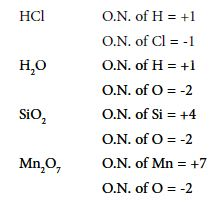# Oxidation Number

Changes in electron distribution are best described using Oxidation Numbers. Oxidation Number (O.N.) is a way of describing how the electron density around an atom within a compound compares to that around the isolated atom. O.N. can be used for ionic or covalently bonded compounds.

Oxidation number of an atom

The Oxidation Number is the modern replacement for ‘valency’. It reflects the ‘combining capacity’ of an element in a compound. The Oxidation Number may be positive or negative. Change in oxidation number reflects whether an atom has been oxidised or reduced as such:

• Positive change = oxidation
• Negative change = reduction

Oxidation numbers do not tell us anything about the type of bonding in a compound. Many elements, particularly non-metals can show more than one O.N.

Rules for assigning O.N.

O.N. of an element in the free or uncombined state is zero. Sum of O.N.s in a neutral molecule or formula unit is zero. In ionic compounds, the O.N. of each element is the charge on its ion. The sum of the O.N.s in a polyatomic ion is equal to the ionic charge of the ion.

Hydrogen normally has O.N. = +1 (except in metal hydrides). Oxygen normally has O.N. = – 2 (except in peroxides and compounds with fluorine). Generally, elements in Group 1 have O.N. +1; and those in Group 2 an O.N. = +2. Chlorine has O.N. = -1 (except in compounds with 0 and F).

In covalent compounds, one element must be given a positive O.N. and the other a negative one. The negative O.N. is assigned to the more non-metallic (more electronegative) atom. It is the hypothetical charge that would result if the electrons in each bond were given to the more electronegative atom.

Example: